International
Tables for
Crystallography
Volume G
Definition and exchange of crystallographic data
Edited by S. R. Hall and B. McMahon

International Tables for Crystallography (2006). Vol. G, ch. 3.4, pp. 137-139

Section 3.4.4. Use of the msCIF dictionary

3.4.4. Use of the msCIF dictionary

| top | pdf |

In this section, some of the capabilities of the dictionary will be demonstrated using simple examples. More detailed examples can be found at http://www.iucr.org/iucr-top/cif/ms and on the CD-ROM accompanying this volume.

3.4.4.1. Description of reciprocal space

| top | pdf |

Modulated and composite structures need more than three reciprocal vectors in order to index the whole set of reflections with integer numbers. Hence a diffraction vector is written as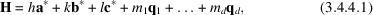where the notation has been chosen according to the core CIF dictionary. In the case of a modulated structure,,andare the reciprocal vectors of the reference structure (and therefore h, k and l index the main reflections).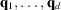are the modulation wave vectors. They are three-dimensional vectors with some irrational component (if the modulated structure is incommensurate) in the lattice spanned by,and. d is the dimension of the modulation. In the case of composite structures, the diffraction pattern can be indexed using 3 + d (arbitrarily selected) vectors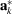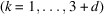.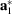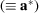,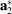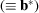and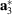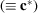normally span the reciprocal lattice of the main reflections of one of the substructures (notice that this is only one particular, but highly intuitive, choice). The remaining d vectors with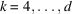are the wave vectors of the modulation [in equation (3.4.4.1)].

In a composite structure, the (3 + d)-dimensional reciprocal basis of the subsystemis determined by a (3 + d) × (3 + d) matrix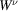[see van Smaalen (1995) and references therein]: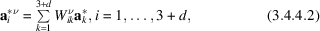where the subscripts i = 1, 2 and 3 label the reciprocal vectors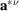,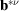and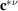, and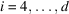label the wave vectors of the modulation expressed as linear combinations of,and.

The simplest case corresponds to a one-dimensional (d = 1) modulated structure. Consider for example the incommensurate phase of K2SeO4. The wave vector of the modulation can be chosen to be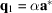. Relevant information about the diffraction pattern of this compound is expressed using both the core CIF and msCIF dictionaries as shown in Example 3.4.4.1.

Example 3.4.4.1. msCIF description of the diffraction pattern of a one-dimensional modulated structure.A more complicated example is the composite structure (LaS)1.14NbS2. The two mutually incommensurate subsystems (along the a axis) are (van Smaalen, 1991) NbS2 (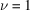) and LaS (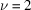). The reciprocal basis can be chosen to be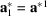,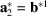,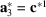and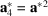. For this particular choice, the two W matrices [see equation (3.4.4.2)] are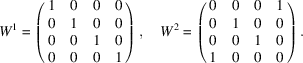This information is transcribed to CIF format as shown in Example 3.4.4.2. (Note that the default values for the wave vector components and the elements of W are 0.)

Example 3.4.4.2. Representation of two mutually incommensurate subsystems.3.4.4.2. Description of symmetry

| top | pdf |

The symmetry of a modulated or composite structure is described by a superspace group which leaves the (3 + d)-dimensional embedding of the structure invariant. Superspace is built of two orthogonal subspaces and both of them are kept invariant separately by the superspace symmetry operations. (In reciprocal space this means that, for these structures, main reflections and satellite reflections are never transformed into one another by superspace symmetry operations.) Consequently, superspace groups are not general (3 + d)-dimensional space groups. The standard notation for superspace groups only covers the one-dimensional superspace groups, which are listed in Janssen et al. (2004). As a consequence, msCIFs must include a list of all the symmetry operations in an (x, y, z) format (using as symbols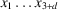) similar to that used in the core CIF dictionary. Superspace-group names for one-dimensional structures can be expressed either according to Janssen et al. (2004) or according to the original notation of de Wolff et al. (1981). Alternative names or higher-dimensional superspace groups can also be included, but not parsed.

In the particular case of K2SeO4, the superspace group is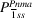(de Wolff et al., 1981) or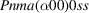(Janssen et al., 2004). This information would appear in a CIF as shown in Example 3.4.4.3.

Example 3.4.4.3. Symmetry description of a superspace group.3.4.4.3. Description of the structure

| top | pdf |

A modulated structure is described by a reference periodic structure and the atomic modulation functions. Such functions are periodic and are normally expanded as Fourier series. The modulated parameters may apply to the atom positions (displacive modulation), the site occupancies (occupational modulation) and/or the temperature factors. In composite structures, each substructure is referred to the crystallographic basis defined by the W matrices [see equation (3.4.4.2)]. The simplest case corresponds to a one-dimensional displacive modulated structure. In this case, the atomic modulation functions are given by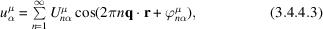where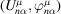is the complex amplitude of each Fourier term;labels the atoms;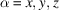; r is the average atom position; and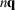represents the successive harmonics of the modulation. At present, displacive modulations along axes other than a, b and c can be calculated with the restriction stated in Section 3.4.2.

Atomic displacements can also be expressed as rigid rotations and translations. The incommensurate phase of K2SeO4 is one-dimensional displacive and sinusoidal (at least over a wide range of temperature), i.e. n = 1 in equation (3.4.4.3). The tetrahedral SeO4 groups behave as rigid bodies. Symmetry considerations restrict the possible translations to occur along c where the only allowed rigid rotations are around the a and b axes. The incommensurate structure is then expressed as shown in Example 3.4.4.4.

Example 3.4.4.4. Atomic displacements as translations and rigid rotations.Alternatively, equation (3.4.4.3) can be expressed as a Fourier series with real amplitudes. This form is also covered by the msCIF dictionary. Note that there is a global phase, which is irrelevant in the incommensurate case but fixes the space group if the modulated structure is commensurate. Global phases are also defined in the dictionary.

3.4.4.4. Block pointers

| top | pdf |

CIFs for modulated and (in particular) composite structures often need several linked data blocks. For example, the average structure of a modulated phase refined using only the main reflections could be reported in one data block and then the results of the refinement of the complete structure using all the reflections could be added as a separate data block at a later stage. Similarly, each substructure in a composite structure can be fully described in a separate data block. It is therefore essential to keep all these blocks together, since all of them together describe the composite structure. Block pointers are described in the core CIF dictionary, but they need to be used with particular care in msCIFs. Therefore, the msCIF dictionary gives additional guidelines for naming the data blocks, as the aim (as in pdCIF) is to define unique names. Example 3.4.4.5shows how the structure of (LaS)1.14NbS2 can be represented using several data blocks. In this example, the block named 1997–07-24|LaSNbS2|G.M.| is linked to those in the loop below.

Example 3.4.4.5. Relationship between data blocks in an msCIF.3.4.4.5. Other information

| top | pdf |

The msCIF and core CIF dictionaries also include items for R factors for different reflection classes (for example, for the main reflections and for the satellite reflections; see Section 3.2.3) and for describing the variation of interatomic distances due to the modulation. msCIF can be used to create a complete record of the determination of a modulated or composite structure suitable for submitting as an article to an academic journal or for archiving in a database. The obvious next step in the development of msCIF is to adapt or write software that can filter and manage msCIF data and that can generate msCIFs automatically.

References

Janssen, T., Janner, A., Looijenga-Vos, A. & de Wolff, P. M. (2004). Incommensurate and commensurate modulated structures. International Tables for Crystallography, Volume C, Mathematical, chemical and physical tables, 3rd ed., edited by E. Prince, ch. 9.8. Dordrecht: Kluwer Academic Publishers.
Smaalen, S. van (1991). Superspace-group approach to the modulated structure of the inorganic misfit layer compound (LaS)1.14NbS2. J. Phys. Condens. Matter, 3, 1247–1263.
Smaalen, S. van (1995). Incommensurate crystal structures. Crystallogr. Rev. 4, 79–202.
Wolff, P. M. de, Janssen, T. & Janner, A. (1981). The superspace groups for incommensurate crystal structures with a one-dimensional modulation. Acta Cryst. A37, 625–636.